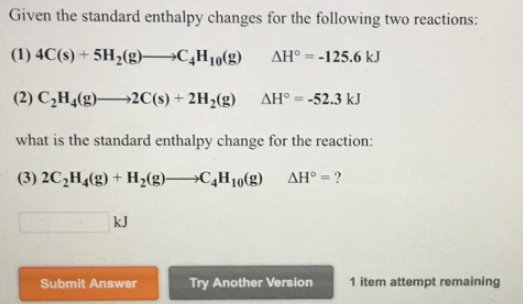# Problem: Given the standard enthalpy changes for the following two reactions: 4C (s) + 5H2 (g) → C4H10 (g)      ΔH° = -125.6 kJ C2H4 (g) → 2C (s) + 2H2 (g)       ΔH° = -52.3 kJ what is the standard enthalpy change for the reaction: 2C2H4 (g) + H2 (g) → C4H10 (g)  ΔH° = ?

🤓 Based on our data, we think this question is relevant for Professor Holland's class at SDSU.

###### FREE Expert Solution###### Problem Details

Given the standard enthalpy changes for the following two reactions:

4C (s) + 5H2 (g) → C4H10 (g)      ΔH° = -125.6 kJ

C2H4 (g) → 2C (s) + 2H2 (g)       ΔH° = -52.3 kJ

what is the standard enthalpy change for the reaction:

2C2H4 (g) + H2 (g) → C4H10 (g)  ΔH° = ?